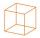# Cube surface area

Wall of cube has content area 99 cm square. What is the surface of the cube?

Result

x =  594 cm2

#### Solution:Leave us a comment of example and its solution (i.e. if it is still somewhat unclear...):

Showing 0 comments:Be the first to comment!## Next similar examples:

1. CubeCalculate the surface cube with edge 11 dm.
2. Cube edgeDetermine the edges of the cube when the surface is equal to 37.5 cm square.
3. The cube - simpleCalculate the surface of the cube measuring 15 centimeters.
4. Cube 2How many times will increase if the surface area of the cube if we triple length of its edge?
5. CubeHow many times increases the surface area of a cube with edge 23.4 cm if the length of the edge doubled?
6. Cube edgesThe sum of the lengths of the cube edges is 42 cm. Calculate the surface of the cube.
7. Cube cornersThe wooden cube with edge 64 cm was cut in 3 corners of cube with edge 4 cm. How many cubes of edge 4 cm can be even cut?
8. Fraction to decimalWrite the fraction 3/22 as a decimal.
9. Forest nurseryIn the forest nursery after winter, they found that 1/10 stems died out of them. For them, they land 193 new spruces. How many spruces are in the forest nursery?
10. Angles 1It is true neighboring angles have not common arm?
11. Mixed2improperWrite the mixed number as an improper fraction. 166 2/3
12. Bus 14Boatesville is 65.35 kilometers from Stanton. A bus traveling from Stanton is 24.13 kilometers from Boatesville. How far has the bus traveled?
13. SimplifySimplify the following problem and express as a decimal: 5.68-[5-(2.69+5.65-3.89) /0.5]
14. Write decimalsWrite in the decimal system the short and advanced form of these numbers: a) four thousand seventy-nine b) five hundred and one thousand six hundred and ten c) nine million twenty-six
15. Addition of Roman numbersAdded together and write as decimal number: LXVII + MLXIV
16. Lengths of the poolMiguel swam 6 lengths of the pool. Mat swam 3 times as far as Miguel. Lionel swam 1/3 as far as Miguel. How many lengths did mat swim?
17. ExpressionSolve for a specified variable: P=a+4b+3c, for a